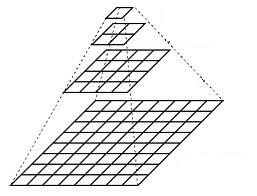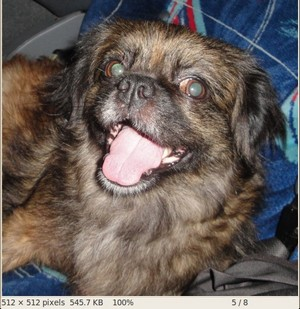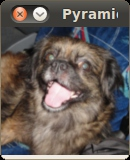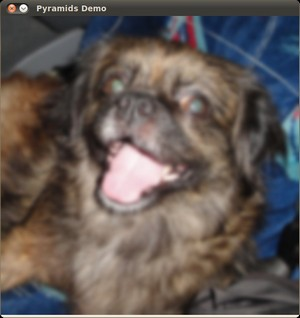OpenCV  3.3.0 Open Source Computer Vision
Image Pyramids

## Goal

In this tutorial you will learn how to:

## Theory

Note
The explanation below belongs to the book Learning OpenCV by Bradski and Kaehler.
• Usually we need to convert an image to a size different than its original. For this, there are two possible options:
1. Upsize the image (zoom in) or
2. Downsize it (zoom out).
• Although there is a geometric transformation function in OpenCV that -literally- resize an image (cv::resize , which we will show in a future tutorial), in this section we analyze first the use of Image Pyramids, which are widely applied in a huge range of vision applications.

### Image Pyramid

• An image pyramid is a collection of images - all arising from a single original image - that are successively downsampled until some desired stopping point is reached.
• There are two common kinds of image pyramids:
• Gaussian pyramid: Used to downsample images
• Laplacian pyramid: Used to reconstruct an upsampled image from an image lower in the pyramid (with less resolution)
• In this tutorial we'll use the Gaussian pyramid.

#### Gaussian Pyramid

• Imagine the pyramid as a set of layers in which the higher the layer, the smaller the size.• Every layer is numbered from bottom to top, so layer $$(i+1)$$ (denoted as $$G_{i+1}$$ is smaller than layer $$i$$ ( $$G_{i}$$).
• To produce layer $$(i+1)$$ in the Gaussian pyramid, we do the following:

• Convolve $$G_{i}$$ with a Gaussian kernel:

$\frac{1}{16} \begin{bmatrix} 1 & 4 & 6 & 4 & 1 \\ 4 & 16 & 24 & 16 & 4 \\ 6 & 24 & 36 & 24 & 6 \\ 4 & 16 & 24 & 16 & 4 \\ 1 & 4 & 6 & 4 & 1 \end{bmatrix}$

• Remove every even-numbered row and column.
• You can easily notice that the resulting image will be exactly one-quarter the area of its predecessor. Iterating this process on the input image $$G_{0}$$ (original image) produces the entire pyramid.
• The procedure above was useful to downsample an image. What if we want to make it bigger?: columns filled with zeros ( $$0$$)
• First, upsize the image to twice the original in each dimension, wit the new even rows and
• Perform a convolution with the same kernel shown above (multiplied by 4) to approximate the values of the "missing pixels"
• These two procedures (downsampling and upsampling as explained above) are implemented by the OpenCV functions cv::pyrUp and cv::pyrDown , as we will see in an example with the code below:
Note
When we reduce the size of an image, we are actually losing information of the image.

## Code

This tutorial code's is shown lines below. You can also download it from here

using namespace cv;
Mat src, dst, tmp;
const char* window_name = "Pyramids Demo";
int main( void )
{
printf( "\n Zoom In-Out demo \n " );
printf( "------------------ \n" );
printf( " * [u] -> Zoom in \n" );
printf( " * [d] -> Zoom out \n" );
printf( " * [ESC] -> Close program \n \n" );
if( src.empty() )
{ printf(" No data! -- Exiting the program \n");
return -1; }
tmp = src;
dst = tmp;
imshow( window_name, dst );
for(;;)
{
char c = (char)waitKey(0);
if( c == 27 )
{ break; }
if( c == 'u' )
{ pyrUp( tmp, dst, Size( tmp.cols*2, tmp.rows*2 ) );
printf( "** Zoom In: Image x 2 \n" );
}
else if( c == 'd' )
{ pyrDown( tmp, dst, Size( tmp.cols/2, tmp.rows/2 ) );
printf( "** Zoom Out: Image / 2 \n" );
}
imshow( window_name, dst );
tmp = dst;
}
return 0;
}

## Explanation

Let's check the general structure of the program:

• Load an image (in this case it is defined in the program, the user does not have to enter it as an argument)
if( src.empty() )
{ printf(" No data! -- Exiting the program \n");
return -1; }
• Create a Mat object to store the result of the operations (dst) and one to save temporal results (tmp).
Mat src, dst, tmp;
/* ... */
tmp = src;
dst = tmp;
• Create a window to display the result
imshow( window_name, dst );
• Perform an infinite loop waiting for user input.
for(;;)
{
char c = (char)waitKey(0);
if( c == 27 )
{ break; }
if( c == 'u' )
{ pyrUp( tmp, dst, Size( tmp.cols*2, tmp.rows*2 ) );
printf( "** Zoom In: Image x 2 \n" );
}
else if( c == 'd' )
{ pyrDown( tmp, dst, Size( tmp.cols/2, tmp.rows/2 ) );
printf( "** Zoom Out: Image / 2 \n" );
}
imshow( window_name, dst );
tmp = dst;
}
Our program exits if the user presses ESC. Besides, it has two options:
• Perform upsampling (after pressing 'u')
if( c == 'u' )
{ pyrUp( tmp, dst, Size( tmp.cols*2, tmp.rows*2 ) );
printf( "** Zoom In: Image x 2 \n" );
}
We use the function cv::pyrUp with three arguments:
• tmp: The current image, it is initialized with the src original image.
• dst: The destination image (to be shown on screen, supposedly the double of the input image)
• *Size( tmp.cols*2, tmp.rows*2 )* : The destination size. Since we are upsampling, cv::pyrUp expects a size double than the input image (in this case tmp).
• Perform downsampling (after pressing 'd')
else if( c == 'd' )
{ pyrDown( tmp, dst, Size( tmp.cols/2, tmp.rows/2 ) );
printf( "** Zoom Out: Image / 2 \n" );
}
Similarly as with cv::pyrUp , we use the function cv::pyrDown with three arguments:
• tmp: The current image, it is initialized with the src original image.
• dst: The destination image (to be shown on screen, supposedly half the input image)
• Size( tmp.cols/2, tmp.rows/2 ) : The destination size. Since we are upsampling, cv::pyrDown expects half the size the input image (in this case tmp).
• Notice that it is important that the input image can be divided by a factor of two (in both dimensions). Otherwise, an error will be shown.
• Finally, we update the input image tmp with the current image displayed, so the subsequent operations are performed on it.
tmp = dst;

## Results

• After compiling the code above we can test it. The program calls an image chicky_512.jpg that comes in the samples/data folder. Notice that this image is $$512 \times 512$$, hence a downsample won't generate any error ( $$512 = 2^{9}$$). The original image is shown below:• First we apply two successive cv::pyrDown operations by pressing 'd'. Our output is:• Note that we should have lost some resolution due to the fact that we are diminishing the size of the image. This is evident after we apply cv::pyrUp twice (by pressing 'u'). Our output is now: